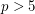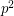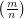# Fibonacci

## Wall-Sun-Sun primes and Fibonacci divisibility ★★

Author(s):

Conjecture   For any prime, there exists a Fibonacci number divisible byexactly once.

Equivalently:

Conjecture   For any prime,does not dividewhereis the Legendre symbol.

Keywords: Fibonacci; prime